## How to sum cells with text and numbers in Excel?

For example, I have a list of values containing numerical and textual strings, now, I want to sum only the numbers based on the same text, look at the following screenshot. Normally, you can’t sum the values in the list with text string directly, here, I will introduce you some formulas to deal with this task.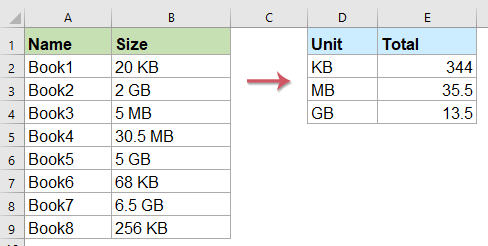#### Sum cells containing text and numbers based on the certain text with formula

You can use an array formula to sum the numbers based on their corresponding text string within the cell, please do as follows:

1. First you can write down your text strings you want to sum the relative numbers in a column cells.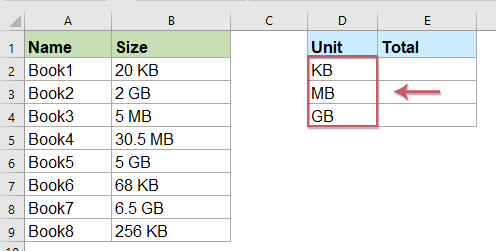2. Then type below formula in a blank cell, in this example, I will enter the formula in cell E2, and then press Ctrl + Shift + Enter keys together, it will return the total value from the list based on the certain text. See screenshot:

=SUM(IF(ISNUMBER(FIND(D2,\$B\$2:\$B\$9)),VALUE(LEFT(\$B\$2:\$B\$9,FIND(D2,\$B\$2:\$B\$9)-1)),0))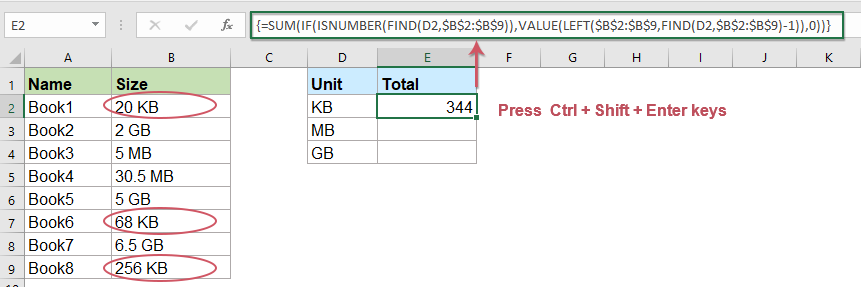Note: In the above formula, D2 is the criterion which you want to use, and B2:B9 indicates the cell range you want to sum.

3. Then select the formula cell, and drag the fill handle down to the cells you want to apply this formula to get the result: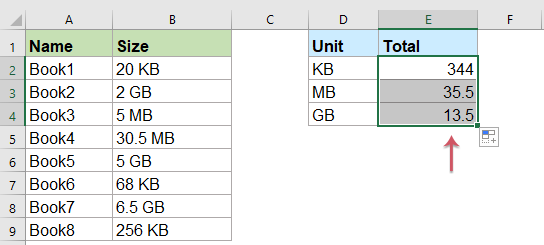#### Sum cells containing text and numbers based on the certain text with a handy feature

If the above formula is hard for you to understand, Kutools for Excel provids a useful tool - Sum based on the same text, with this feature, you can get the total result within a cell which mixed with numbers and text without remembering any formulas.

Note:To apply these Sum based on the same text features, firstly, you should download the Kutools for Excel, and then apply the features quickly and easily.

After installing Kutools for Excel, please do as follows:

1. Click to select a cell where you want to output the result, see screenshot:

Tips: To prevent circular references, please don’t insert this formula into the first row of the worksheet.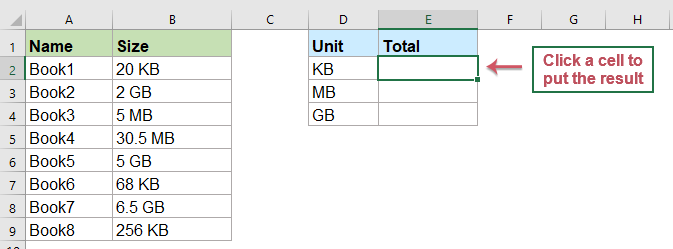2. And then click Kutools > Formula Helper > Formula Helper, see screenshot: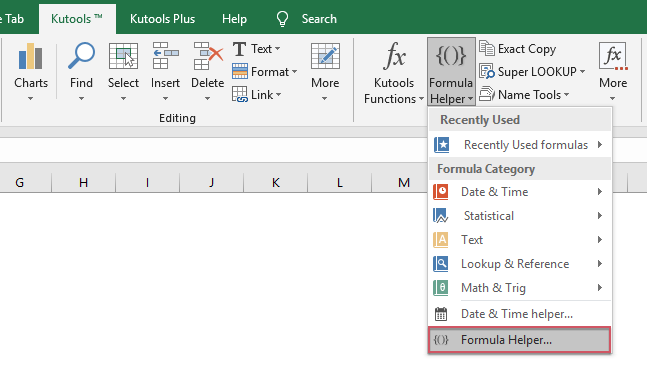3. In the Formulas Helper dialog box, please do the following operations:

• Select Statistical from the Formula Type drop down list;
• In the Choose a formula list box, click to select Sum based on the same text option;
• Then, in the Arguments input section, select the range of cells containing the text and numbers that you want to sum in the Range textbox, and then, select the text cell you want to sum values based on in the Text textbox.
Tips: In the Text textbox, you should change the default absolute cell reference to relative cell reference for dragging the formula correctly.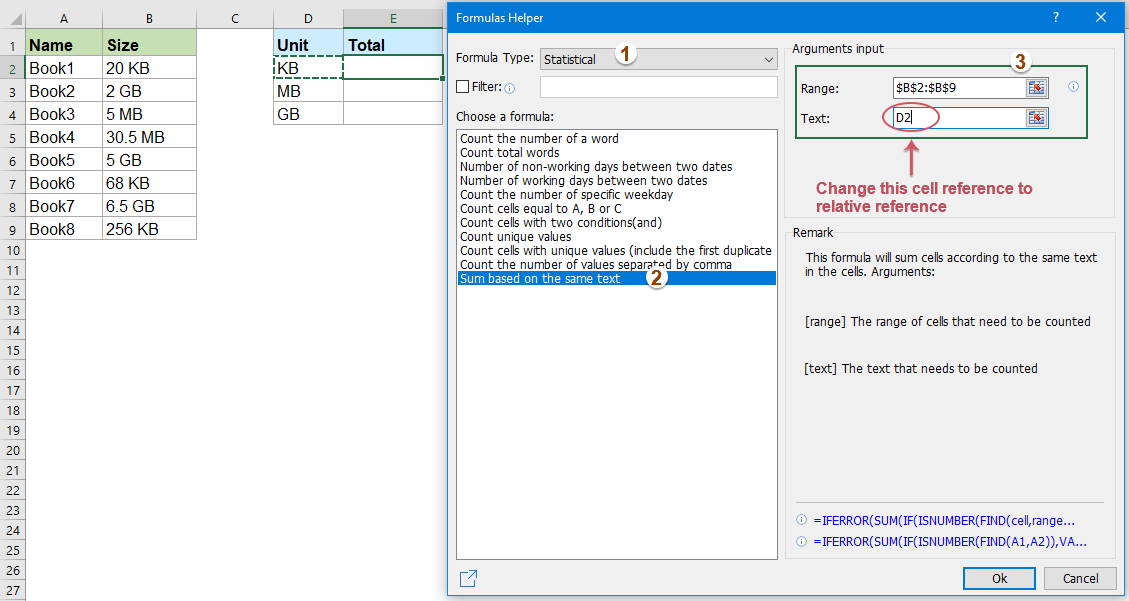4. And then, click Ok button, the first result will be calculated, then drag the fill handle for fill the formula to other cells, see screenshot: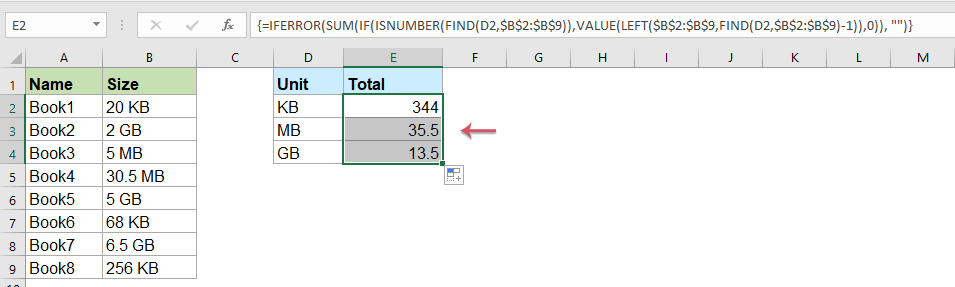#### Sum cells containing text and numbers ignoring the text with User Defined Function

If you have the following data which is mixed with text and numbers, and you just want to extract and add up only the numbers in each cell, how could you quickly finish this in Excel?.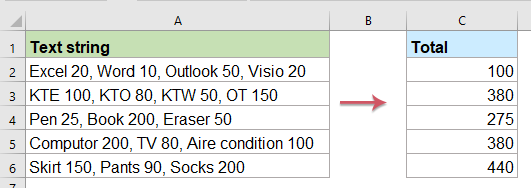To deal with this problem, you can create a User Defined Function, please do as this:

1. Hold down the ALT + F11 keys to open the Microsoft Visual Basic for Applications window.

2. Click Insert > Module, and paste the following code in the Module Window.

VBA code: sum only the numbers in the cell containing numbers and text

``````Function SumNumbers(rngS As Range, Optional strDelim As String = " ") As Double
'Updateby Extendoffice
Dim xNums As Variant, lngNum As Long
xNums = Split(rngS, strDelim)
For lngNum = LBound(xNums) To UBound(xNums) Step 1
SumNumbers = SumNumbers + Val(xNums(lngNum))
Next lngNum
End Function
``````

3. Then save and close this code, go back to the worksheet, and enter this formula =SumNumbers(A2) (A2 indicates the cell you want to sum up the numbers), then drag the fill handle down to the cells you want to fill the formula, and only numbers in each cell are added together, see screenshot: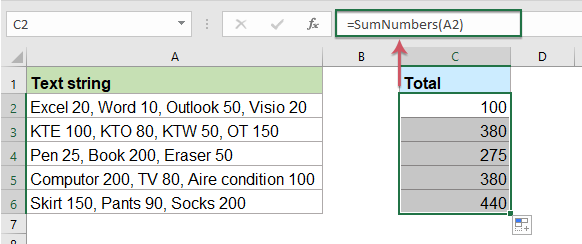#### More relative articles:

• Combine Duplicate Rows And Sum The Values In Excel
• In Excel，you may always meet this problem, when you have a range of data which contains some duplicate entries, and now you want to combine the duplicate data and sum the corresponding values in another column, as following screenshots shown. How could you solve this problem?
• Multiply Two Columns And Then Sum In Excel
• In Excel, most of us may suffer to multiply two columns and then add them up, of course, we can multiply each items and then sum them, but this will be troublesome if there are hundreds or thousands rows need to calculate. In Excel, there is a powerful function – SUMPRODUCT, with it, we can quickly multiply two columns and then sum them. This article, I will talk about how to apply this function.
• Sum Top 3 Or N Values Based On Criteria In Excel
• Normally, we can sum the top n values from a range of cells by using a simple formula, but have you ever tried to sum top n values based on some criteria. This article, I will introduce some formulas for summing top n values with specific criteria in Excel.
• Sum Unique Values Based On Criteria In Excel
• For example, I have a range of data which contains Name and Order columns, now, to sum only unique values in Order column based on the Name column as following screenshot shown. How to solve this task quickly and easily In Excel?
• Count Or Sum Cells Based On The Font Colors In Excel
• How could you get the number of cells or sum all the cells which contain a particular font color in Excel? For example, I have a range of data in a worksheet as following screenshot shown, and now I want to respectively count or sum the cells that have a red, blue and black font color. Normally, there is no direct way to deal with this task, here, I will talk about some tricks for soling this job.

### Best Office Productivity Tools

#### Supercharge Your Spreadsheets：Experience Efficiency Like Never Before with Kutools for Excel

 Popular Features: Find/Highlight/Identify Duplicates   |  Delete Blank Rows   |  Combine Columns or Cells without Losing Data   |   Round without Formula ... Super Lookup: Multiple Criteria VLookup  |   Multiple Value VLookup  |   VLookup Across Multiple Sheets   |   Fuzzy Lookup .... Advanced Drop-down List: Quickly Create Drop Down List   |  Dependent Drop Down List   |  Multi-select Drop Down List .... Column Manager: Add a Specific Number of Columns   |   Move Columns   |   Unhide Columns   |   Compare Columns to Select Same & Different Cells ... Featured Features: Grid Focus   |  Design View   |   Big Formula Bar   |  Workbook & Sheet Manager   |  Resource Library (Auto Text)   |  Date Picker   |  Combine Worksheets   |  Encrypt/Decrypt Cells   |  Send Emails by List   |  Super Filter   |   Special Filter (filter bold/italic/strikethrough...) ... Top 15 Toolset:  12 Text Tools (Add Text, Remove Characters, ...)   |   50+ Chart Types (Gantt Chart, ...)   |   40+ Practical Formulas (Calculate age based on birthday, ...)   |   19 Insertion Tools (Insert QR Code, Insert Picture from Path, ...)   |   12 Conversion Tools (Numbers to Words, Currency Conversion, ...)   |   7 Merge & Split Tools (Advanced Combine Rows, Split Cells, ...)   |   Many More...

Kutools for Excel boasts over 300 features, ensuring that what you need is just a click away...

Supports Office/Excel 2007-2021 & newer, including 365   |   Available in 44 languages   |   Enjoy a full-featured 30-day free trial.#### Office Tab Brings Tabbed interface to Office, and Make Your Work Much Easier

• Enable tabbed editing and reading in Word, Excel, PowerPoint, Publisher, Access, Visio and Project.
• Open and create multiple documents in new tabs of the same window, rather than in new windows.
• Increases your productivity by 50%, and reduces hundreds of mouse clicks for you every day!No ratings yet. Be the first to rate!
This comment was minimized by the moderator on the site
Hi, I am trying to check approximately 8 different types of leave. I am using conditional formatting to colour code letters and then calculate as numbers. It works well, I like it. I did not factor in a half day.

Can anyone tell me how to account for a half day, and still have Excel recognize the Alpha symbol.
This comment was minimized by the moderator on the site
Hi, how do I sum something like the following:
Column A
Row 1 2D
Row 2 2N
Row 3 1D+2N

I have no issues summing the Ds but when I try summing the Ns I would encounter a problem. The string 1D+2N is the culprit, so how do I go about this?
Any help is very much appreciated.

Thank you.
This comment was minimized by the moderator on the site
I must be doing something incorrect. When I try to use the SumNumbers() VB code nothing happens? excel does not recognize it as code? I am using a macro enabled document. Image attached.
https://ibb.co/6vRtD6N

[img]https://ibb.co/6vRtD6N[/img]
This comment was minimized by the moderator on the site
Hi, Michael,
I have tested the code based on the data you provied, it can work well.
This is User Defined Function, so, you needn't run the code, you just need to insert the formula SumNumbers(A1), and press Enter key to get the result. See screenshot: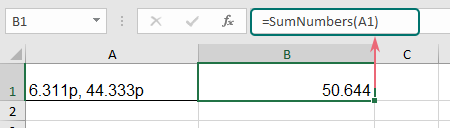This comment was minimized by the moderator on the site
How to do this, but with decimal places? When using decimals, it returns 0...
This comment was minimized by the moderator on the site
HOW TO MULTIPLY COLUM 1 , 2 PCS , COLOUM 2 RATE 1 USD TOTAL AMOUNT ?
This comment was minimized by the moderator on the site
kindly reply to "I want to use this formula to sum only numbers in all columns like =SumNumbers(A1:G1) but it gives error VALUE. how it can be fixed?"
This comment was minimized by the moderator on the site
You need to use the VBA for that to work. the VBA code defines the SumNumbers as a function.
This comment was minimized by the moderator on the site
I have used VBA for that but it doesn't work. Can you plz take a look?
This comment was minimized by the moderator on the site
I have used VBA for that but it does not work. Can you take a look plz?
This comment was minimized by the moderator on the site
I want to use this formula to sum only numbers in all columns like =SumNumbers(A1:G1) but it gives error VALUE. how it can be fixed?
This comment was minimized by the moderator on the site
I am using this in excel 2016 but it doesn't seem to be working is there a work through? i keep getting a "
#name?" error
This comment was minimized by the moderator on the site
Hi,
I have a question. How to get the sum or the average of the column that has text inside that column. Please see attachment. Your answer would be greatly appreciated.
This comment was minimized by the moderator on the site
Apple 10 (+)
Apple 5 (-)
Apple 20 (+)
Apple 15 (+)
Apple 30 (+)
Apple 25 (-)

Can anyone help solve this?
This comment was minimized by the moderator on the site
I have a variant of this problem you solved with the SumNumbers module. The issue is that the cell contains both dollar values and other numbers, for example:
"Case of Soda (354mL x 24): USD 25.00 Case of Water (0.500L x 24): USD 15.00"

Using the SumNumbers module adds up all numbers but we only want the dollar values.
This comment was minimized by the moderator on the site
If you have the following data which is mixed with text and numbers, and you just want to extract and add up only the numbers in each cell, how could you quickly finish this in Excel?.

Bro, but I only want to sum specific number after specific word? can I do that?
This comment was minimized by the moderator on the site
Thank you very much!!!
This comment was minimized by the moderator on the site
In this formula =SUM(IF(ISNUMBER(FIND(D1,\$B\$2:\$B\$8)),VALUE(LEFT(\$B\$2:\$B\$8,FIND(D1,\$B\$2:\$B\$8)-1)),0)) can be put a range in place of D1 - say D1:D5 - not getting result, if i put it this way. What is the correct way to do this?
This comment was minimized by the moderator on the site
Hi, I want to be able to add a number and also text to one cell (C5 all the way to C16) then get only the numbers in there to add up. But the =SumNumbers(A1) formula doesn't seem to work.
This comment was minimized by the moderator on the site
Hi, I hope someone can help. I like to add inside the cell +100j+50f+600m but must display/add on cell as 750 only. So you see 750 in cell but when press F2, it will show =100j+50f+600m
This comment was minimized by the moderator on the site
Hi. Please can someone help me. I am trying to SUM a column in EXCEL that has numbers and letters. eg 8.1km, 10.5km etc. There are 1031 lines or cells in the column. I am using this formula =SUM(LEFT(D3),LEN(D3)-2+LEFT(D4),LEN(D4)-2) which works if i want to add 2 or 3 cells together but i cant use this for 1031 cells. i wanted to add a : in place of the + but it comes back with an error and will not allow me to create the formula. Any help will be greatly appreciated
This comment was minimized by the moderator on the site
Hi,
How to multiply 10 pcs *200,

not 10*200
This comment was minimized by the moderator on the site
10 pcs *200
10 pcs in cell A1 and 200 in cell B1
then enter below formula in cell C1
=SUBSTITUTE(A1," pcs","")*B1
This comment was minimized by the moderator on the site
Hi,

Thank you for this post! I am keen on using the VBA code and I was thinking if it would be possible to have a multivariable function so that you can sum the numbers from different column cells and write something like SumNumbers(A1:C1), for example.

Many Thanks

Giovanni
This comment was minimized by the moderator on the site
Hello, Giovanni,
Can you give an example of your problem?
You can attach a screenshot here.
Thank you!
This comment was minimized by the moderator on the site
I am also looking to see if this function can sum multiple cells.
This comment was minimized by the moderator on the site
=SumNumbers(D8:AH8)
This comment was minimized by the moderator on the site
hi can anyone help me how to add below numbers with letters? i tried to do like but it doest work i think i miss something in the formula=SUM(IF(ISNUMBER(FIND(D1,\$B\$2:\$B\$8)),VALUE(LEFT(\$B\$2:\$B\$8,FIND(D1,\$B\$2:\$B\$8)-1)),0)). please help.Thanks.

2L
5D
10S
3L
11D
16S
This comment was minimized by the moderator on the site
Hello, Anna,
First, you should extract the letters into another helper column, and then apply the above formula, after entering this formula, please remember to press Shift + Ctrl + Enter keys together. see the following screenshot image:
This comment was minimized by the moderator on the site
Hi There! I have two types of numbers in one column, one is numeric numbers, the other is a character followed by a number (such as n20, n30, etc). I wish to add up those numbers with an "n". I applied your guidance for "Sum Cells Containing Text And Numbers Based On The Certain Text With Formula", but it did not work. Is there any other method? Thanks in advance!
This comment was minimized by the moderator on the site
April May June July Total 1100 / 65658 1200/65657 1500/65656 2100/65650 ? i want to total only 1100+1200+1500+2100 Other not a value only Receipt no. please help any
This comment was minimized by the moderator on the site
How To Sum of selected word in cell in excel Exam 1100/656480 1100 is a amount and 656480 is recpt. no. but i want to total only 1100
This comment was minimized by the moderator on the site
Hello, The =SUM(IF(ISNUMBER(FIND(D1,\$B\$2:\$B\$8)),VALUE(LEFT(\$B\$2:\$B\$8,FIND(D1,\$B\$2:\$B\$8)-1)),0)) - worked perfectly, except that my text is on the right. I tried to change the formula however that is not working... i just keep getting #VALUE! Can anyone help?
This comment was minimized by the moderator on the site
Remember to press Ctrl+Shift+Enter after changing the formula, not just Enter.
This comment was minimized by the moderator on the site
Can anyone help me with a sum formula? I'm trying to sum a column with cells that contain both numbers and text. I want to sum the numbers to the left of the text letter. sample: 3P30 2P30 2P30 2P30 1P20 3P30 1P20 3P50 3P30 3P60
This comment was minimized by the moderator on the site
How to include decimals in the "=SumNumbers(A1)" formula? Thank you!
This comment was minimized by the moderator on the site
Here's a simple script that accepts single dimension and 2 dimensional selection range of cells with each cell containing string or numbers but with each cell reduced to single numbers and returns the sum of all cells. Option Explicit Function SUMCELLS(InputRange As Range) As Double Dim Arr() As Variant Arr = InputRange Dim R As Long Dim C As Long SUMCELLS = 0 For R = 1 To UBound(Arr, 1) ' First array dimension is rows. For C = 1 To UBound(Arr, 2) ' Second array dimension is columns. Debug.Print Arr(R, C) Dim NumericValue As Double Dim StringValue As String StringValue = Trim(CStr(Arr(R, C))) If (Not (StringValue = "")) Then NumericValue = ONLYDIGITS(StringValue) SUMCELLS = SUMCELLS + NumericValue End If Next C Next R End Function Function ONLYDIGITS(s As String) As String Dim retval As String Dim i As Integer Dim periods As Integer periods = 0 retval = "" ' For i = 1 To Len(s) Dim char As String char = Mid(s, i, 1) If (char >= "0" And char
This comment was minimized by the moderator on the site
I was able to use this formula perfectly, but I would also like to add a condition to only include certain numbers in the SUM formula if the value in another column = "defects". My original formula (using your original post) is: =SUM(IF(ISNUMBER(FIND("hrs",E17:E38)),VALUE(LEFT(E17:E38,FIND("hrs",E17:E38)-1)),0)) Where E17:E38 contains the text and numbers that I want to sum And B17:B38 contains the values "features" and "defects" How can I adjust the formula to only SUM those numbers in column E if the value in column B = "defects"? Thanks in advance! Kimberly,
This comment was minimized by the moderator on the site
How to convert between text and number using KUTOOls ?
This comment was minimized by the moderator on the site
Kutools
Content
Number to word
This comment was minimized by the moderator on the site
GENUIS.... Thanks ever so much
This comment was minimized by the moderator on the site
This is super helpful! I'm trying to get the ARRAY to work with a column of cells that each contains a decimal number and text (e.g. .2 A; .5 C; .1 X etc), and I keep getting a result of 0. If I change the numbers to whole numbers, the result is correct. Help?
This comment was minimized by the moderator on the site
is the any way to rewrite the vba code to sum multi cells instead of one? I have about 8 columns of data and i want the sum at the end.
This comment was minimized by the moderator on the site
Is it possible to change the SumNumbers formula to add all the numbers in a column of mixed cells? For example, each column has 1A, 2B, 3C and I would like the formula at the bottom of the column to tell me 6.
This comment was minimized by the moderator on the site
The SumNumbers macro saved me many hours of manual work. Thank you!
This comment was minimized by the moderator on the site
ok, i understood this. good luck.
This comment was minimized by the moderator on the site
In A1 Cell of excel I input data as like 5+6+3+1-2 And A2 Cell of excel give the Result 13 how it possible in excel.
This comment was minimized by the moderator on the site
insert "space" before "+" and "-"
This comment was minimized by the moderator on the site
i think you are real teacher and good man. good luck.
There are no comments posted here yet